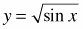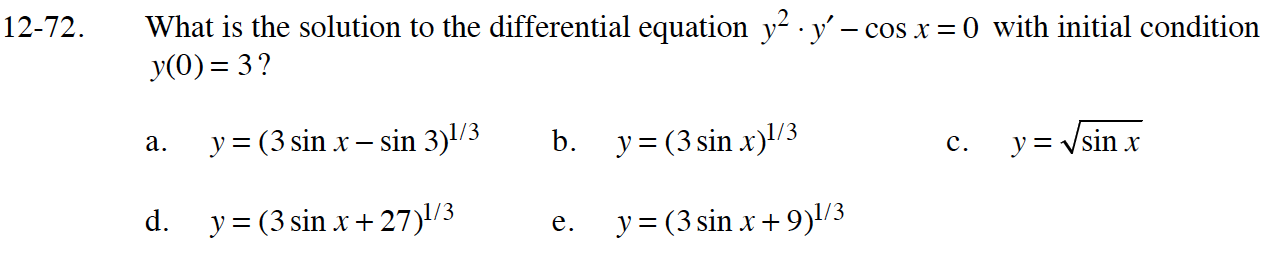### Home > CALC > Chapter 12 > Lesson 12.2.1 > Problem12-72

12-72.
1. What is the solution to the differential equation y2 · y' − cos x = 0 with initial condition y(0) = 3? Homework Help ✎

1. y = (3 sin x sin 3)1/3

2. y = (3 sin x)1/3

3.4. y = (3 sin x + 27)1/3

5. y = (3 sin x + 9)1/3After integrating both sides:

Use (0, 3) to solve for the value of C. Then write the equation in y = form.